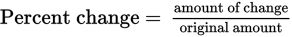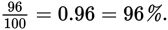# SAT Math Multiple Choice Question 415: Answer and Explanation

### Test Information

Question: 415

10. In an effort to decrease reliance on fossil fuels, some energy producers have started to utilize renewable resources. One such power plant uses solar panels to create solar energy during the day and then shifts to natural gas at night or when there is cloud cover. One particularly bright morning, the company increases the amount of its power typically generated by solar panels by 60%. During a cloudy spell, it decreases the amount by 30%, and then when the sun comes back out, it increases the amount again by 75% before shutting the panels down for the night. What is the net percent increase of this company's reliance on solar panels during that day?

• A. 75%
• B. 96%
• C. 105%
• D. 165%

Getting to the Answer: Whenever you aren't given a concrete starting point, pick one yourself. The best number to use when dealing with percents is 100. First, find how much power was derived from the solar panels after the first increase: 100 × 0.6 = 60. So, the company increased power from the solar panels to 100 + 60 = 160. Next, find the amount after the decrease: 160 × 0.3 = 48, so the solar panels then provided 160 - 48 = 112 units of power. Finally, find the amount after the last increase: 112 × 0.75 = 84, so the plant ended the day at 112 + 84 = 196, which is 196 - 100 = 96 more than it started the day with. To find the percent change, use the formulato get